MATHGOTSERVED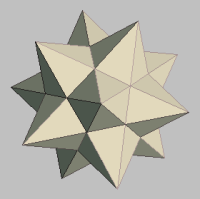PRECALCULUS
UNIT 3: EXPONENTS AND LOGARITHMS
3-1 EXPONENTIAL FUNCTIONS AND GRAPHS
Exponential Function
An exponential function can be described as a function with a variable exponent and a numerical base.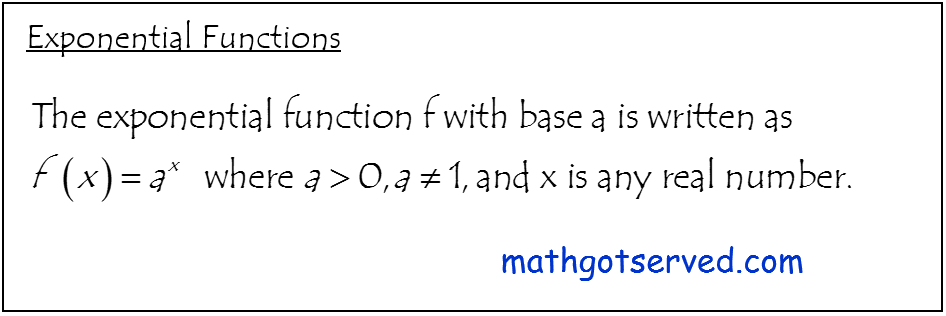How to Graph Exponential Functions
Property of Exponents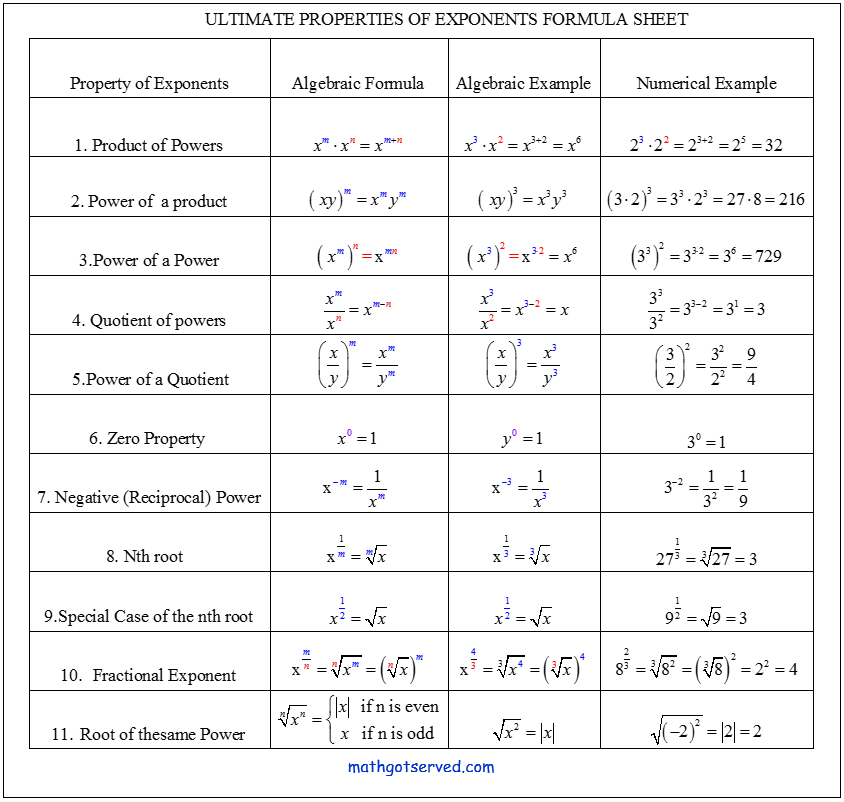3-2 LOGARITHMIC FUNCTIONS & GRAPHS
Logarithmic  Function
the The Logarithmic function can be thought of as the inverse of an exponential function with the same base as the logarithmic function. Logarithmic functions have many applications one main use is in solving exponential equations. Logs were also very important in the past in accelerating computation of products, quotients and powers.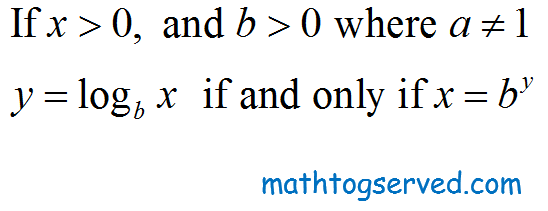How to Graph Logarithmic Functions
Properties of Logarithms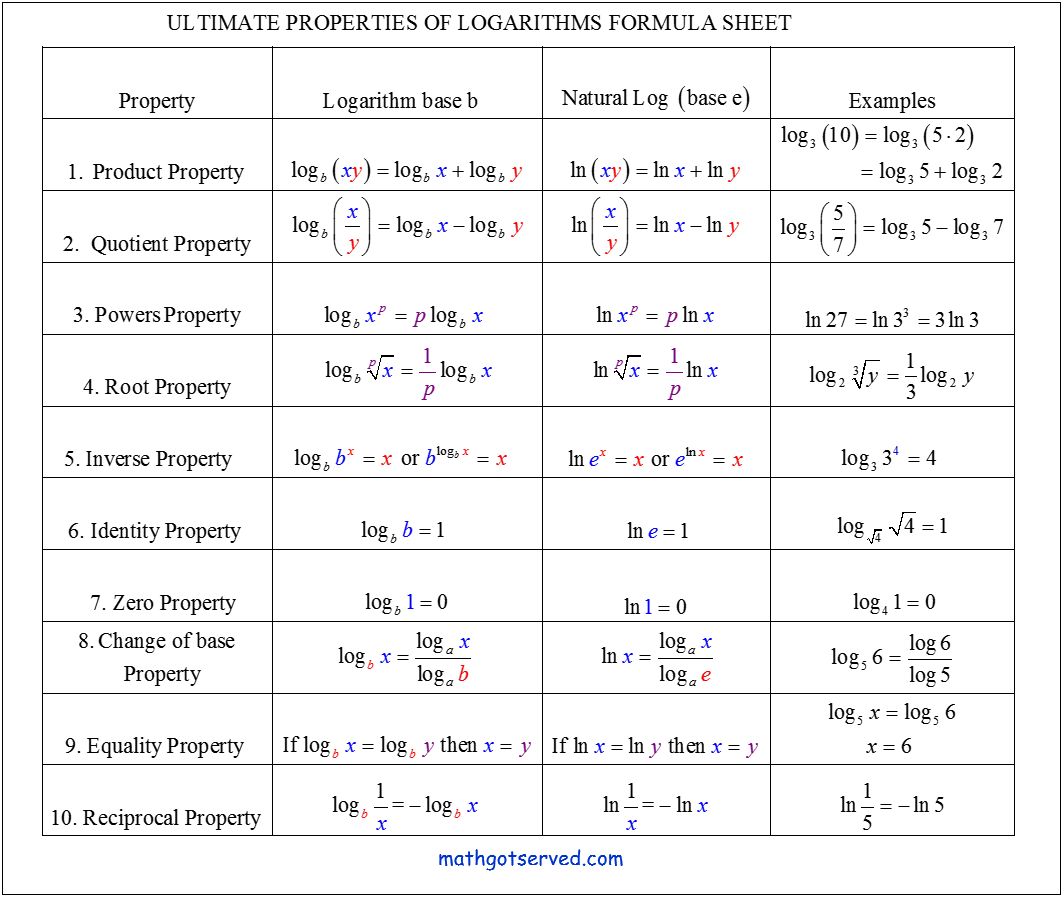1-3 GRAPHS OF FUNCTION AND ITS PROPERTIES
Definition  (Meaning) of a Domain and Range:
Graphically the domain can be described as the horizontal span of the graph of a function when it is projected on the x-axis. The domain can be thought of as the width of the graph of a function. The range on the other hand is the vertical span of the graph of a function when it is projected on the y-axis. It can be thought of as the height of a function.

VIDEO TUTORIAL ON LOGARITHMS